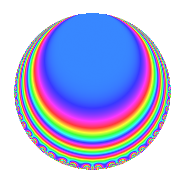# Properties

 Label 1224.1.mLevel 1224 Weight 1 Character orbit m Rep. character $$\chi_{1224}(305,\cdot)$$ Character field $$\Q$$ Dimension 4 Newform subspaces 2 Sturm bound 216 Trace bound 5

# Related objects

## Defining parameters

 Level: $$N$$ $$=$$ $$1224 = 2^{3} \cdot 3^{2} \cdot 17$$ Weight: $$k$$ $$=$$ $$1$$ Character orbit: $$[\chi]$$ $$=$$ 1224.m (of order $$2$$ and degree $$1$$) Character conductor: $$\operatorname{cond}(\chi)$$ $$=$$ $$51$$ Character field: $$\Q$$ Newform subspaces: $$2$$ Sturm bound: $$216$$ Trace bound: $$5$$

## Dimensions

The following table gives the dimensions of various subspaces of $$M_{1}(1224, [\chi])$$.

Total New Old
Modular forms 28 4 24
Cusp forms 12 4 8
Eisenstein series 16 0 16

The following table gives the dimensions of subspaces with specified projective image type.

$$D_n$$ $$A_4$$ $$S_4$$ $$A_5$$
Dimension 0 0 4 0

## Trace form

 $$4q + O(q^{10})$$ $$4q - 4q^{13} - 4q^{19} + 4q^{43} - 4q^{49} + 4q^{55} + 4q^{85} + O(q^{100})$$

## Decomposition of $$S_{1}^{\mathrm{new}}(1224, [\chi])$$ into newform subspaces

Label Dim. $$A$$ Field Image CM RM Traces $q$-expansion
$$a_2$$ $$a_3$$ $$a_5$$ $$a_7$$
1224.1.m.a $$2$$ $$0.611$$ $$\Q(\sqrt{-2})$$ $$S_{4}$$ None None $$0$$ $$0$$ $$-2$$ $$0$$ $$q-q^{5}-\beta q^{7}-q^{11}-q^{13}-q^{17}+\cdots$$
1224.1.m.b $$2$$ $$0.611$$ $$\Q(\sqrt{-2})$$ $$S_{4}$$ None None $$0$$ $$0$$ $$2$$ $$0$$ $$q+q^{5}-\beta q^{7}+q^{11}-q^{13}+q^{17}+\cdots$$

## Decomposition of $$S_{1}^{\mathrm{old}}(1224, [\chi])$$ into lower level spaces

$$S_{1}^{\mathrm{old}}(1224, [\chi]) \cong$$ $$S_{1}^{\mathrm{new}}(204, [\chi])$$$$^{\oplus 4}$$

## Hecke characteristic polynomials

$p$ $F_p(T)$
$2$ 1
$3$ 1
$5$ ($$( 1 + T + T^{2} )^{2}$$)($$( 1 - T + T^{2} )^{2}$$)
$7$ ($$1 + T^{4}$$)($$1 + T^{4}$$)
$11$ ($$( 1 + T + T^{2} )^{2}$$)($$( 1 - T + T^{2} )^{2}$$)
$13$ ($$( 1 + T + T^{2} )^{2}$$)($$( 1 + T + T^{2} )^{2}$$)
$17$ ($$( 1 + T )^{2}$$)($$( 1 - T )^{2}$$)
$19$ ($$( 1 + T + T^{2} )^{2}$$)($$( 1 + T + T^{2} )^{2}$$)
$23$ ($$( 1 - T + T^{2} )^{2}$$)($$( 1 + T + T^{2} )^{2}$$)
$29$ ($$( 1 + T^{2} )^{2}$$)($$( 1 + T^{2} )^{2}$$)
$31$ ($$1 + T^{4}$$)($$1 + T^{4}$$)
$37$ ($$1 + T^{4}$$)($$1 + T^{4}$$)
$41$ ($$( 1 + T + T^{2} )^{2}$$)($$( 1 - T + T^{2} )^{2}$$)
$43$ ($$( 1 - T + T^{2} )^{2}$$)($$( 1 - T + T^{2} )^{2}$$)
$47$ ($$1 + T^{4}$$)($$1 + T^{4}$$)
$53$ ($$1 + T^{4}$$)($$1 + T^{4}$$)
$59$ ($$( 1 - T )^{2}( 1 + T )^{2}$$)($$( 1 - T )^{2}( 1 + T )^{2}$$)
$61$ ($$1 + T^{4}$$)($$1 + T^{4}$$)
$67$ ($$( 1 + T^{2} )^{2}$$)($$( 1 + T^{2} )^{2}$$)
$71$ ($$( 1 + T^{2} )^{2}$$)($$( 1 + T^{2} )^{2}$$)
$73$ ($$1 + T^{4}$$)($$1 + T^{4}$$)
$79$ ($$( 1 - T )^{2}( 1 + T )^{2}$$)($$( 1 - T )^{2}( 1 + T )^{2}$$)
$83$ ($$1 + T^{4}$$)($$1 + T^{4}$$)
$89$ ($$1 + T^{4}$$)($$1 + T^{4}$$)
$97$ ($$( 1 - T )^{2}( 1 + T )^{2}$$)($$( 1 - T )^{2}( 1 + T )^{2}$$)